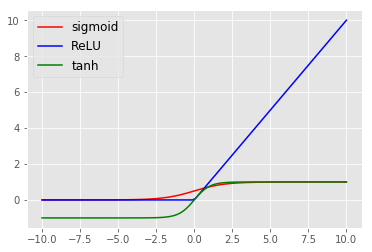In :
def sigmoid(z):
return 1./(1+np.exp(-z))

def ReLU(z):
return np.maximum(0,z)

def tanh(z):
return np.tanh(z)

z = np.linspace(-10,10,100)
plt.plot(z, sigmoid(z),'r', label = 'sigmoid')
plt.plot(z, ReLU(z),'b',label ='ReLU')
plt.plot(z, tanh(z),'g',label ='tanh')
plt.legend(fontsize=12)

Out:
<matplotlib.legend.Legend at 0x18df61fb390>In :
x1 = np.linspace(-2,2,50)
x2 = np.linspace(-2,2,50)
x3 = np.linspace(-1,1,50*50)

result = np.zeros((50*50,3))

out11 = np.zeros((50*50, 1))
out12 = np.zeros((50*50, 1))
out13 = np.zeros((50*50, 1))
out14 = np.zeros((50*50, 1))

out21 = np.zeros((50*50, 1))
out22 = np.zeros((50*50, 1))
out23 = np.zeros((50*50, 1))
out24 = np.zeros((50*50, 1))
out25 = np.zeros((50*50, 1))
out26 = np.zeros((50*50, 1))

out31  = np.zeros((50*50, 1))

for i in range(len(x1)):
for j in range(len(x2 )):
# Input layer
n11 = x1[i] * weights0 + x2[j] * weights0 + biases0
a11 = tanh(n11)

n12 = x1[i] * weights0 + x2[j] * weights0 + biases0
a12 = tanh(n12)

n13 = x1[i] * weights0 + x2[j] * weights0 + biases0
a13 = tanh(n13)

n14 = x1[i] * weights0 + x2[j] * weights0 + biases0
a14 = tanh(n14)

# Hidden layer
n21 = a11 * weights1 + a12 * weights1 + a13 * weights1 + a14 * weights1 + biases1
a21 = ReLU(n21)

n22 = a11 * weights1 + a12 * weights1 + a13 * weights1 + a14 * weights1 + biases1
a22 = ReLU(n22)

n23 = a11 * weights1 + a12 * weights1 + a13 * weights1 + a14 * weights1 + biases1
a23 = ReLU(n23)

n24 = a11 * weights1 + a12 * weights1 + a13 * weights1 + a14 * weights1 + biases1
a24 = ReLU(n24)

n25 = a11 * weights1 + a12 * weights1 + a13 * weights1 + a14 * weights1 + biases1
a25 = ReLU(n25)

n26 = a11 * weights1 + a12 * weights1 + a13 * weights1 + a14 * weights1 + biases1
a26 = ReLU(n26)

# Output layer

n31 = a21 * weights2 + a22 * weights2 \
+ a23 * weights2 + a24 * weights2 \
+ a25 * weights2 + a26 * weights2 \
+ biases2
a31 = sigmoid(n31)

result[i*50+j,0 ] = x1[i]
result[i*50+j, 1] = x2[j]

out11[i*50+j] = a11
out12[i*50+j] = a12
out13[i*50+j] = a13
out14[i*50+j] = a14

out21[i*50+j] = a21
out22[i*50+j] = a22
out23[i*50+j] = a23
out24[i*50+j] = a24
out25[i*50+j] = a25
out26[i*50+j] = a26

out31[i*50+j] = a31